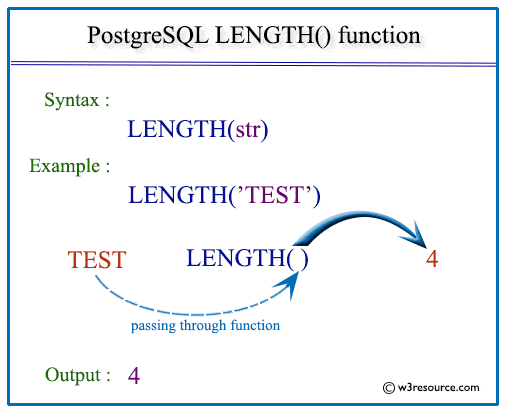# PostgreSQL LENGTH() function

## LENGTH() function

The PostgreSQL length() function is used to find the length of a string i.e. number of characters in the given string.

Syntax:

`length(<string>)`

PostgreSQL Version: 9.3

Pictorial Presentation of PostgreSQL LENGTH() functionExample: PostgreSQL LENGTH() function:

In the example below the length function returns the length of the given string 'w3resource'.

Code:

``````SELECT length('w3resource')
AS "Length of a String";
```
```

Sample Output:

``` Length of a String
--------------------
10
(1 row)
```

Example of PostgreSQL LENGTH() function using column :

Sample Table: employees

The example below, returns the first_name and the length of first_name ( how many characters contain in the first name ) from the employees where the length of first_name is more than 7.

Code:

``````SELECT first_name,length(first_name)
AS "Length of a First Name"
FROM employees
WHERE length(first_name)>7;
```
```

Sample Output:

``` first_name  | Length of a First Name
-------------+------------------------
Alexander   |                      9
Jose Manuel |                     11
Alexander   |                      9
Christopher |                     11
Danielle    |                      8
Harrison    |                      8
Elizabeth   |                      9
Jonathon    |                      8
Kimberely   |                      9
Jennifer    |                      8
Jennifer    |                      8
(11 rows)
```

Previous: LEFT function